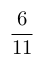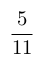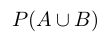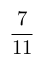Select Page

# MCQ in English Answers for CBSE Maths 12 Science Probability

MCQ in English Answers for CBSE Maths 12 Science Probability to enable students to get Answers in a narrative video format for the specific question.

Expert Teacher provides MCQ Answers for CBSE Maths 12 Science Probability through Video Answers in English language. This video solution will be useful for students to understand how to write an answer in exam in order to score more marks. This teacher uses a narrative style for a question from Probability not only to explain the proper method of answering question, but deriving right answer too.

Please find the question below and view the Answer in a narrative video format.

Question:

## Similar Questions from CBSE, 12th Science, Maths, Probability

Question 1 : A die is thrown 6 times. If 'getting an odd number' is a 'success', what is the probability of obtaining:
atleast 5 successes?

Question 2 : A bag A contains 4 black and 6 red balls and bag B 7 black and 3 red balls. A die is thrown. If 1 or 2 appears on it, then bag A is chosen, otherwise bag B. It two balls are drawn at random (without replacement) from the selected bag, find the probability of one of them being red and another black.        (View Answer Video)

Question 3 :  In a group of 400 people, 160 are smokers and non-vegetarian, 100 are smokers and vegetarian and the remaining are non-smokers and vegetarian. The probabilities of getting a special chest disease are 35%, 20% and 10% respectively. A person is chosen from the group at random and is found to be suffering from the disease. What is the probability that the selected person is a smoker and non-vegetarian? What value is reflected in this question?     (View Answer Video)

Question 4 : Ifandthen find P(E / F). (View Answer Video)

Question 5 : If P(A)=, P(B)=and=, find. (View Answer Video)

### Relations and Functions

Question 1 : A functiondefined asis, (View Answer Video)

Question 2 : Let * be the binary operation on N given by a * b = LCM of a and b. Find 5 * 7. (View Answer Video)

Question 3 :  If f(x) = ax + b and g(x) = cx + d, then f[g(x)] – g[f(x)] is equivalent to, (View Answer Video)

Question 4 : Let * be the binary operation on N given by a * b = LCM of a and b. Find 5 * 7. (View Answer Video)

Question 5 : Let A = {1, 2, 3}. Then, number of relations containing (1, 2) and (1, 3) which are reflexive and symmetric but not transitive is, (View Answer Video)

### Three Dimensional Geometry

Question 1 : Find the equation of the plane passing through the line of intersection of the planeandwhich is at a unit distance from the origin. (View Answer Video)

Question 2 : If a line makes angleswith the positive direction of co-ordinate axes, then write the value of(View Answer Video)

Question 3 : Write the sum of intercepts cut off by the planeon the three axes.  (View Answer Video)

Question 4 : Find the distance between the point (-1, -5, -10) and the point of intersection of lineand plane

Question 5 : Find the co-ordinates of the point where the line through the points A (3, 4, 1) and B (5, 1, 6) crosses the XY-plane. (View Answer Video)

### Continuity and Differentiability

Question 1 : Findfor the function. (View Answer Video)

Question 2 : Findfor the function. (View Answer Video)

Question 3 : Differentiate w.r.t.x the function, for some constant a and b. (View Answer Video)

Question 4 : Differentiate the function w.r.t.x. (View Answer Video)

Question 5 : Findfor the function. (View Answer Video)